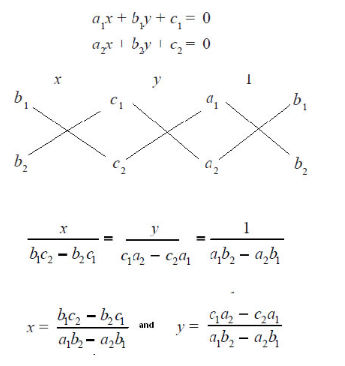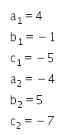# Solve the system of equation by cross multiplication method

solve the system of equation by cross multiplication method
4x-y=5; 5y-4x=7